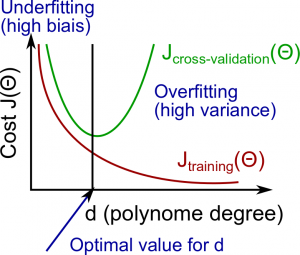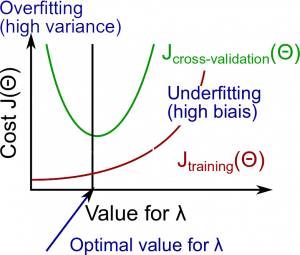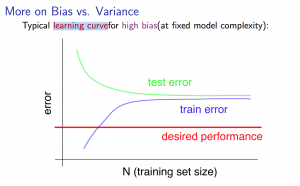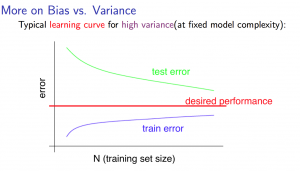Categories:

Updated:

# Model Selection and Train / Cross Validation / Test Set

How should we select the best model for the problem?

The first idea is to train each model on the entire dataset that you have and compare the mean squared errors.

However, if we evaluate and train on the same dataset, our model will “overfit”; the parameter theta will try too hard to fit that specific dataset, thus not generalize well to other datasets.

The second idea is to split the dataset into train set and cross validation set. Then we train each model on the train set and evaluate it based on the cross validation set. We choose model that performs best on cross validation set.

However, the score itself is overfitted to the cross validation set, since we choose hyperparameters such as regularization term lambda based on that specific cross validation set. So the score evaluates the model too optimistically.

The third idea is to split the dataset into train, cross validation, and test set. We fit parameters like theta with the train set, hyperparameters like lambda with the cross validation set, and score the selected model based on the test set.

One example way to break down our dataset into the three sets is;

• Training set: 60%
• Cross Validation Set: 20%
• Test set: 20%

# Bias vs Variance

Let’s assume that we want to choose the hyperparameter ‘d’, which is polynomial degree of polynomial regression.

The training error will tend to decrease as we increase the degree d of the polynomial.

At the same time, the cross validation error will tend to decrease as we increase d up to a point, and then it will increase as d is increased, forming a convex curve.

High bias (underfitting)

both $J_{train}(\Theta)$ and $J_{CV}(\Theta)$ will be high. Also, $J_{CV}(\Theta) \approx J_{train}(\Theta)$

High variance (overfitting)

$J_{train}(\Theta)$ will be low and $J_{CV}(\Theta)$ will be much greater than $J_{train}(\Theta)$.

The is represented in the figure below:In general, complex model is sensitive to data, so it is much affected by changes in input. Therefore it has high variance and low bias.

Also, simple model is more rigid to input changes, thus has low variance and high bias.

# Regularization and Bias/Variance

The relationship of λ to the training set and the variance set is as follows:

Low $\lambda$

$J_{train}(\Theta)$ is low and $J_{CV}(\Theta)$ is high (high variance/overfitting).

Intermediate $\lambda$

$J_{train}(\Theta)$ and $J_{CV}(\Theta)$ are somewhat low and $J_{train}(\Theta) \approx J_{CV}(\Theta)$.

Large $\lambda$

both $J_{train}(\Theta)$ and $J_{CV}(\Theta)$ will be high (underfitting /high bias).# Training Set Size and Bias/Variance (Learning Curve)

With high bias

• Low training set size: causes $J_{train}(\Theta)$ to be low and $J_{CV}(\Theta)$ to be high.

• Large training set size: causes both $J_{train}(\Theta)$ and $J_{CV}(\Theta)$ to be high with $J_{train}(\Theta) \approx J_{CV}(\Theta)$.

If a learning algorithm is suffering from high bias, getting more training data will not (by itself) help much.With high variance

• Low training set size: $J_{train}(\Theta)$ will be low and $J_{CV}(\Theta)$ will be high.

• Large training set size: $J_{train}(\Theta)$ increases with training set size and $J_{CV}(\Theta)$ continues to decrease without leveling off. Also, $J_{train}(\Theta) < J_{CV}(\Theta)$ but the difference between them remains significant.

If a learning algorithm is suffering from high variance, getting more training data will help.# Solving Bias and Variance

### Solving Bias(Underfitting)

• Decreasing lambda
• Increasing number of layers and units in neural network
• Decreasing dropout prob in neural network
• Increasing polynomial degree in polynomial regression
• Other methods to increase complexity in a particular model

### Solving Variance(Overfitting)

• Fetching more data
• Trying smaller sets of features
• Increasing lambda
• Decreasing number of layers and units in neural network
• Increasing dropout prob in neural network
• Introducing batchnormalization in neural network
• Decreasing polynomial degree in polynomial reagression
• Other methods to decrease model complexity

Categories: